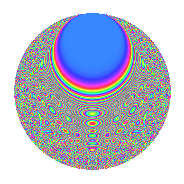# Properties

 Label 8038.2.aLevel 8038 Weight 2 Character orbit a Rep. character $$\chi_{8038}(1,\cdot)$$ Character field $$\Q$$ Dimension 334 Newform subspaces 4 Sturm bound 2010 Trace bound 1

# Related objects

## Defining parameters

 Level: $$N$$ $$=$$ $$8038 = 2 \cdot 4019$$ Weight: $$k$$ $$=$$ $$2$$ Character orbit: $$[\chi]$$ $$=$$ 8038.a (trivial) Character field: $$\Q$$ Newform subspaces: $$4$$ Sturm bound: $$2010$$ Trace bound: $$1$$

## Dimensions

The following table gives the dimensions of various subspaces of $$M_{2}(\Gamma_0(8038))$$.

Total New Old
Modular forms 1007 334 673
Cusp forms 1004 334 670
Eisenstein series 3 0 3

The following table gives the dimensions of the cuspidal new subspaces with specified eigenvalues for the Atkin-Lehner operators and the Fricke involution.

$$2$$$$4019$$FrickeDim.
$$+$$$$+$$$$+$$$$84$$
$$+$$$$-$$$$-$$$$83$$
$$-$$$$+$$$$-$$$$92$$
$$-$$$$-$$$$+$$$$75$$
Plus space$$+$$$$159$$
Minus space$$-$$$$175$$

## Trace form

 $$334q + 2q^{3} + 334q^{4} - 2q^{5} - 4q^{7} + 336q^{9} + O(q^{10})$$ $$334q + 2q^{3} + 334q^{4} - 2q^{5} - 4q^{7} + 336q^{9} + 4q^{11} + 2q^{12} - 4q^{13} + 16q^{15} + 334q^{16} + 4q^{17} - 2q^{19} - 2q^{20} + 10q^{22} - 20q^{23} + 336q^{25} - 2q^{26} - 4q^{27} - 4q^{28} - 12q^{29} - 8q^{31} + 16q^{33} + 4q^{34} + 4q^{35} + 336q^{36} + 4q^{37} + 8q^{38} + 16q^{39} - 4q^{41} - 30q^{43} + 4q^{44} + 22q^{45} + 4q^{46} + 8q^{47} + 2q^{48} + 314q^{49} - 16q^{50} + 16q^{51} - 4q^{52} - 14q^{53} + 12q^{54} + 20q^{55} - 16q^{57} - 6q^{58} + 12q^{59} + 16q^{60} - 28q^{61} - 4q^{62} + 4q^{63} + 334q^{64} + 28q^{65} - 34q^{67} + 4q^{68} + 56q^{69} - 8q^{70} - 4q^{71} - 24q^{73} - 6q^{74} + 46q^{75} - 2q^{76} + 20q^{77} - 44q^{78} + 28q^{79} - 2q^{80} + 374q^{81} - 16q^{82} - 6q^{83} - 40q^{85} + 12q^{86} + 52q^{87} + 10q^{88} + 32q^{89} + 8q^{90} - 20q^{92} + 48q^{93} + 16q^{95} - 24q^{97} + 40q^{99} + O(q^{100})$$

## Decomposition of $$S_{2}^{\mathrm{new}}(\Gamma_0(8038))$$ into newform subspaces

Label Dim. $$A$$ Field CM Traces A-L signs $q$-expansion
$$a_2$$ $$a_3$$ $$a_5$$ $$a_7$$ 2 4019
8038.2.a.a $$75$$ $$64.184$$ None $$75$$ $$-30$$ $$-29$$ $$-31$$ $$-$$ $$-$$
8038.2.a.b $$83$$ $$64.184$$ None $$-83$$ $$20$$ $$31$$ $$-3$$ $$+$$ $$-$$
8038.2.a.c $$84$$ $$64.184$$ None $$-84$$ $$-19$$ $$-32$$ $$1$$ $$+$$ $$+$$
8038.2.a.d $$92$$ $$64.184$$ None $$92$$ $$31$$ $$28$$ $$29$$ $$-$$ $$+$$

## Decomposition of $$S_{2}^{\mathrm{old}}(\Gamma_0(8038))$$ into lower level spaces

$$S_{2}^{\mathrm{old}}(\Gamma_0(8038)) \cong$$ $$S_{2}^{\mathrm{new}}(\Gamma_0(4019))$$$$^{\oplus 2}$$

## Hecke characteristic polynomials

There are no characteristic polynomials of Hecke operators in the database